# AP Calculus AB Practice Test 42

### Test Information9 questions18 minutes

Calculator Disallowed

1. As the tides change, the water level in a bay varies sinusoidally. At high tide todayat 8 A.M., the water level was 15 feet; at low tide, 6 hours later at 2 P.M., it was 3 feet. How fast, in feet per hour, was the water level dropping at noontoday?

2. A smooth curve with equation y = f (x) is such that its slope at each x equals x2. If the curve goes through the point (-1, 2), then its equation is

3.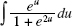is equal to

4. Given f (x) = log10x and log10(102)2.0086, which is closest to f (100)?

5. If G(2) = 5 and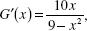then an estimate of G(2.2) using a tangent-line approximation is

6. The area bounded by the parabola y = x2 and the lines y = 1 and y = 9 equals

7. Suppose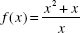if x ≠ 0 and f (0) = 1. Which of the following statements is (are) true of f ?

I. f is defined at x = 0.

II.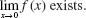III. f is continuous at x = 0.

8. Which function could have the graph shown below?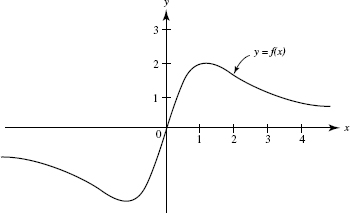9. Suppose the graph of f is both increasing and concave up on axb. Then, using the same number of subdivisions, and with L, R, M, and T denoting, respectively, left, right, midpoint, and trapezoid sums, it follows that